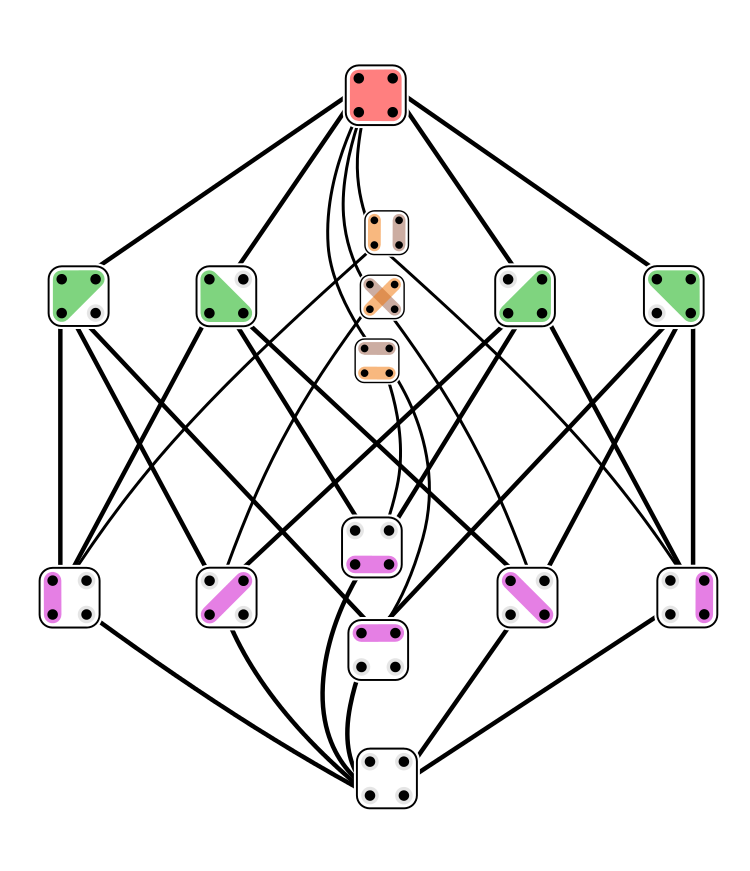Great! These pictures depict **[partitions](https://en.wikipedia.org/wiki/Partition_of_a_set)** of the set {1,2,3,4,5,6}: that is, ways of writing it as a disjoint union of nonempty subsets called **blocks**.

The collection of all partitions of a set is partially ordered by 'refinement': we say one partition \\(P\\) is **finer** than another partition \\(P'\\) if every block of \\(P'\\) is a union of blocks of \\(P\\). (One could say "finer than or equal to", but people say just "finer".) We also say, in this situation, that \\(P'\\) is **coarser** than \\(P\\).

We write \\(P \le P'\\) if \\(P\\) is finer than \\(P'\\).

Here's a picture of all 15 partitions of a 4-element set, with finer ones nearer the bottom, and edges pointing up from each partition to the next coarser ones. This is called a [Hasse diagram](https://en.wikipedia.org/wiki/Hasse_diagram):The picture was drawn by [Tilman Piesk](https://commons.wikimedia.org/wiki/File:Set_partitions_4;_Hasse;_circles.svg) on Wikicommons. Click on it for a lot more information.

For any two partitions \\(P,P'\\) of a set \\(S\\), their **meet** \\(P \vee P'\\) is the finest partition that is coarser than both. That's what this problem is about.